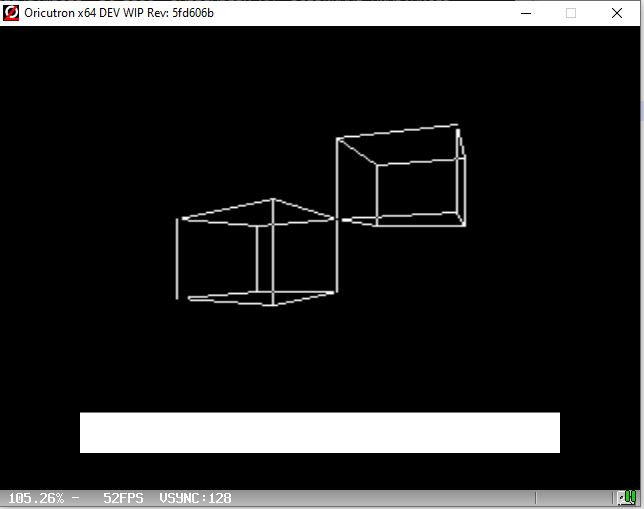Page 1 of 1

### Hyper Fast 3D Projection Routine for your Real Time Apps

Posted: Mon Dec 23, 2019 2:02 pm
Hi every body,

My name is Jean-Baptiste.

I'm a developer on glOric project at Oric Software and I want to inform oric'developers that a very nice function was recently pushed on the gloric repository at Oric Software.

It is what could be called an "Hyper Fast 3D Projection Routine" named `glProject`.

This function is of interest for people intending to render 3D scene on Oric in real time context because it computes screen coordinates from 3D coordinates very fast (about 500 cycles per point).

It is not yet totally packaged but here's its SoW:

Given the camera situation (position and orientation) and a screen configuration, this function gives screen 2d-coordinates of up to 64 projected 3d points.

Code: Select all

``````// Camera Position
extern int CamPosX;
extern int CamPosY;
extern int CamPosZ;

// Camera Orientation
extern char CamRotZ;
extern char CamRotX;

void glProject (char points2D[], char points3D[], unsigned char nbPoints);``````

As input of the function, array `points3D` contains list of `nbPoints` 3D points stored on 4 bytes: 3 bytes for X, Y and Z coordinates and a spare bytes for futur use.

As output of function, array `points2D` if filled with `nbPoints` 2D points stored on 4 bytes: 2 bytes for L and C (line and column coordinates) and 2 bytes for distance (between camera and point).

The 2d-coordinates thus obtained can either be used to simply display points on screen, draw lines and faces between them, or be used as base coordinates for drawing tiles.

For exemple, here's a piece of code showing how to project a 3D cube:

Code: Select all

``````#include <glOric.h>

#define CUBE_SIZE	4
#define NB_POINTS_CUBE		8

const char ptsCube3D[]={
-CUBE_SIZE, -CUBE_SIZE, +CUBE_SIZE, 0 // P0
,-CUBE_SIZE, -CUBE_SIZE, -CUBE_SIZE, 0 // P1
,+CUBE_SIZE, -CUBE_SIZE, -CUBE_SIZE, 0 // P2
,+CUBE_SIZE, -CUBE_SIZE, +CUBE_SIZE, 0 // P3
,-CUBE_SIZE, +CUBE_SIZE, +CUBE_SIZE, 0 // P4
,-CUBE_SIZE, +CUBE_SIZE, -CUBE_SIZE, 0 // P5
,+CUBE_SIZE, +CUBE_SIZE, -CUBE_SIZE, 0 // P6
,+CUBE_SIZE, +CUBE_SIZE, +CUBE_SIZE, 0 // P7
};
char ptsCube2D [NB_POINTS_CUBE	* SIZEOF_2DPOINT];

glProject (points2D, points3D, NB_POINTS_CUBE);
``````
after the call to `glProject` array `points2D` contains 2D coordinates of points in `points3D`

Provided you have a function `drawLine (X1, Y1, X2, Y2)` that draws a segment between points [X1, Y1] and [X2, Y2], it is possible to draw the cube with following instructions:

Code: Select all

``````void lineBetweenPoints (idxPt1, idxPt2 ){
drawLine (
// L , C Point 1
ptsCube2D [idxPt1*SIZEOF_2DPOINT + 0],
ptsCube2D [idxPt1*SIZEOF_2DPOINT + 1],
// L , C Point 2
ptsCube2D [idxPt2*SIZEOF_2DPOINT + 0],
ptsCube2D [idxPt2*SIZEOF_2DPOINT + 1]
);
}

lineBetweenPoints (0, 1);
lineBetweenPoints (1, 2);
lineBetweenPoints (2, 3);
lineBetweenPoints (3, 0);
lineBetweenPoints (4, 5);
lineBetweenPoints (5, 6);
lineBetweenPoints (6, 7);
lineBetweenPoints (7, 4);
lineBetweenPoints (0, 4);
lineBetweenPoints (1, 5);
lineBetweenPoints (2, 6);
lineBetweenPoints (3, 7);
``````
```
The code available on glOric repository is under development, but two little demo are provided on this thread.